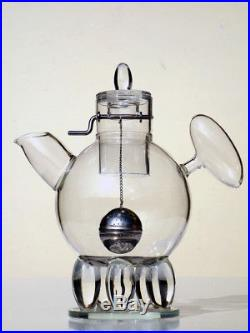# MICHAEL GRAVES rare glass teapot Nanna design Leonardo with box” N A N N A “. M i c h a e l G r a v e s. L e o n a r d o , 1 9 9 6. G l a s s T e a p o t. O r i g i n a l b o x. P e r f e c t C o n d i c t i o n. M E A S U R E S. 1 1 – 1 / 2 x 1 0 x 5 – 1 / 2 i n c h. W E S H I P I N W O R L D W I D E. S H I P. I N G C O S T. I T A L Y. E U R O P E. O T H E R. C O U N T R Y. P l e a s e. T E R M S O F S A L E. P A Y M E N T. P a y p a l – B a n k T r a n s f e r. P R O F E S S I O N A L P A C K E T. B u b b l e W r a p – P o l y s t y r e n e c u b e s – H a r d C a r d b o a r d B o x e s. C O M M U N I C A T I O N. P r o m p t a n s w e r w i t h i n 2 4 h o u r s. I t e m 1 0 0. The item “MICHAEL GRAVES rare glass teapot Nanna design Leonardo with box” is in sale since Tuesday, September 26, 2017. This item is in the category “Antiques\Periods & Styles\Mid-Century Modernism”. The seller is “tangram_xy07″ and is located in Milano. This item can be shipped worldwide.
• Maker: Leonardo
• Material: Glass
• MEASURES: 11-1/2 x 10 x 5 -1/2 in.